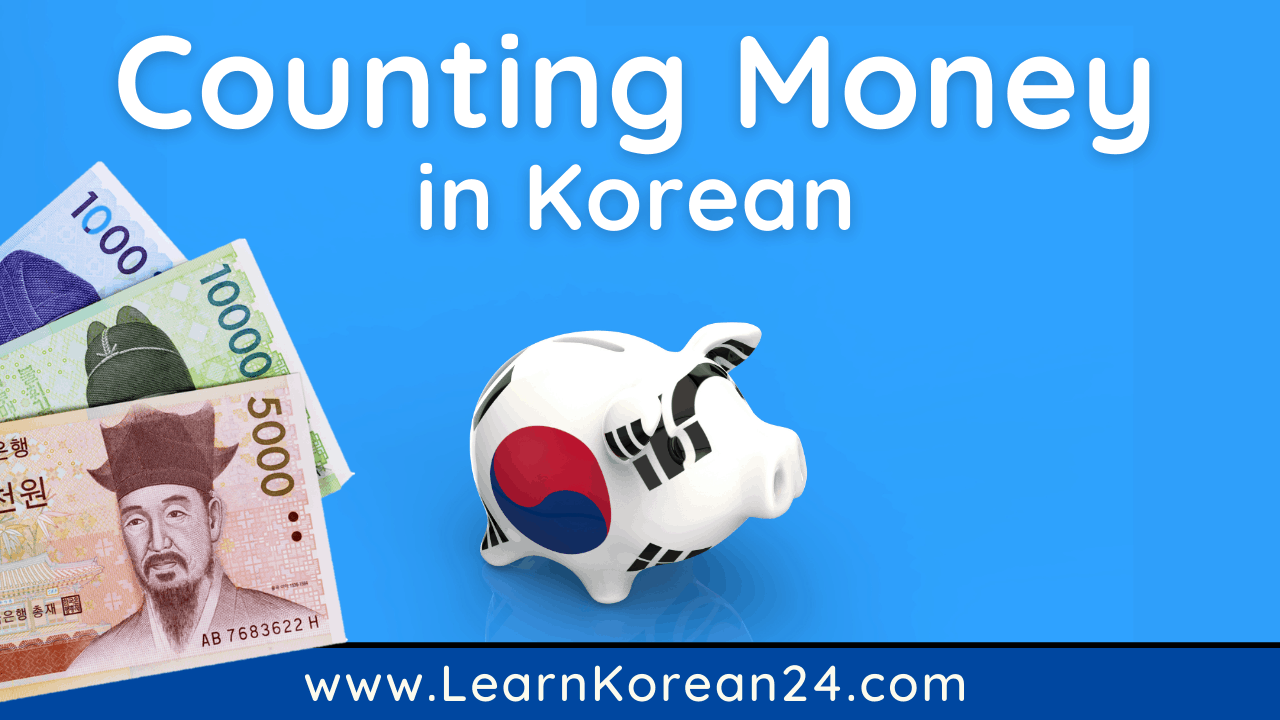# Money In Korean – How To Count Korean MoneyIf you are learning Korean or intend to visit Korea, learning about money in Korean is essential. When visiting stores, restaurants, coffee shops, buying train/bus tickets, etc, you will inevitably have to talk about price and how much things cost. To do so, you need to know about money in Korean and how to count Korean money. In this post, you will learn how to talk about the Korean currency and how to count Korean money.

## Money In Korean

The word for ‘money’ in Korean is [don]. In order to talk about money in Korean, you need to know the Korean currency, the common Korean currency denominations, and Sino-Korean numbers. Let’s look at each of these individually before looking at some examples of counting money in Korean.

### Korean Currency

The currency in Korea is the [won]. Technically, each [won] can be divided into 10 [jeon], but this is such a small amount that there are no jeon coins circulating in Korea today. In fact, the smallest denomination of Korean won these days is 10원. So, in order to talk about Korean money, you only need to remember the word [won].

The symbol for the Korean 원 [won] is ₩. When writing the price of something in Korean the symbol ₩ comes before the number, and when saying the price you simply say 원 [won] after the number. So, for example, ten Korean won would be written as ₩10 and spoken as 십 원 [sip won].

### Common Korean Currency Denominations

#### Coins

As was mentioned above, the smallest denomination of the Korean currency you will find in circulation in Korea today is the 10원 coin. Next is the 50원 coin, then the 100원 coin, followed by the 500원 coin.

#### Notes

There are just 4 denominations of Korean notes. These are 1000원, 5000원, 10,000원, and 50,000원.

### Sino-Korean Numbers

There are two number systems in Korea which are Sino-Korean numbers and Native Korean numbers. When counting money in Korean, Sino-Korean numbers are used and so to count money in Korean you need to know Sino-Korean numbers. Luckily, these numbers are really easy to learn and you only need to learn 18 words to be able to count to 1 billion in Korean. If you’ve not learned Sino-Korean numbers yet, check out our complete guide to Korean numbers.

Here are the Sino-Korean numbers together the Korean currency 원 [won] for the Korean currency denominations:

## How To Count Money In Korean

Now that you know the Korean currency (원) and the common Korean currency denominations, it’s really easy to count money in Korean if you know Sino-Korean numbers. To say any amount of money in Korean, you simply say the number using Sino-Korean numbers followed by the word 원 [won]. Here are some example sentences using ‘How much is it?’ in Korean.

## Practice Exercise

Practice counting money in Korean with this fun quiz. You will be asked ‘How much is it?’ and then you must choose the correct price in Korean.

Are you ready to get serious about learning Korean? Start our 100% FREE online Korean language course today!